4WX Internet Search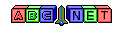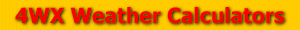# Heat Index

From the user, we are given an air temperature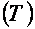and a relative humidity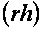.

If the air temperature is given in degrees Celsius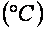, we must convert the temperature value to degrees Fahrenheit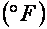To do this, check out the temperature conversion formula at:

Then, we can calculate the heat index with this complex formula: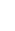Back to 4WX Weather Calculators index page

4WX Calculators Credit and disclaimer